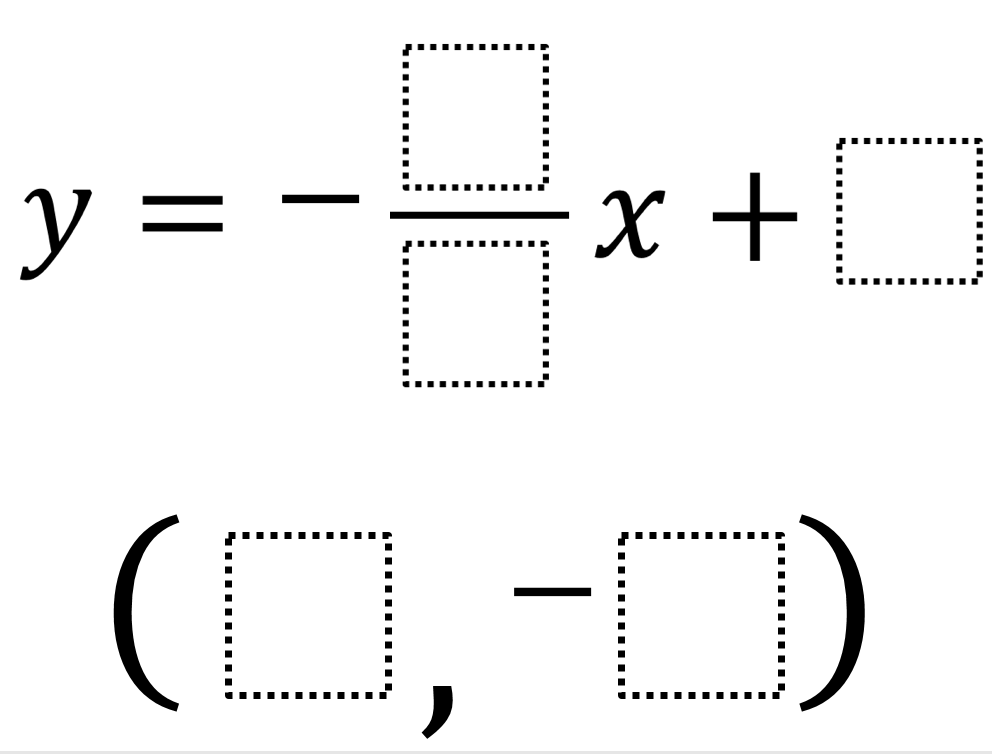# Max Intercept

Directions: Using the digits 1 to 9 at most one time each, fill in the boxes to write the equation of a line that passes through the point with the largest possible y-intercept. How many solutions can you find?### Hint

For a given y-intercept, how do you figure out a possible slope and point?

### Answer

17 possible solutions that have 9 as the y-intercept:
y=-8/1x+9 through (2, -7)
y=-7/1x+9 through (2, -5)
y=-6/1x+9 through (2, -3)
y=-5/1x+9 through (3, -6)
y=-8/2x+9 through (4, -7)
y=-3/1x+9 through (5, -6)
y=-6/2x+9 through (4, -3)
y=-4/2x+9 through (5, -1)
y=-6/3x+9 through (5, -1)
y=-8/4x+9 through (5, -1)
y=-2/1x+9 through (6, -3)
y=-4/2x+9 through (6, -3)
y=-8/4x+9 through (6, -3)
y=-2/1x+9 through (7, -5)
y=-4/2x+9 through (7, -5)
y=-6/3x+9 through (7, -5)
y=-8/4x+9 through (7, -5)

Source: Andy Schwen

## Minimize Slope

Directions: Given the point (3,5), use digits 1-9, at most one time, to find a …

### 5 comments

1.Shouldn’t there be a negative with the slope? Also the title says maximum slope, but the directions do not mention that.

2.Yes. This was very confusing. I did not want to look at the answer but then I figured out the slope MUST be negative for this to work. the directions don’t allow for a negative number:(.

3.The slope is now showing as negative, and the “max” has been limited to just the y-intercept.